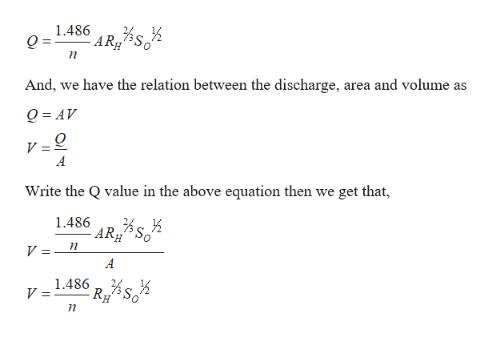# Water flows in a rectangular flume 15 ft wide made of brick with cement mortar (n = 0.015). Find the depth of the water under uniform flow at 18 fps with a slope of 0.02. What would be the resulting error in the estimated depth if the “wide-and-shallow” flow assumption were used?

Question
6 views

Water flows in a rectangular flume 15 ft wide made of brick with cement mortar (n = 0.015). Find the depth of the water under uniform flow at 18 fps with a slope of 0.02. What would be the resulting error in the estimated depth if the “wide-and-shallow” flow assumption were used?

check_circle

Step 1

Given information:

For the rectangular section b=15ft

Manning’s coefficient n= 0.015

Volume V = 18fps.

Slope S= 0.02

Step 2

Calculation:

We have the manning’s formulahelp_outlineImage Transcriptionclose1.486 AR And, we have the relation between the discharge, area and volume as Q AV V=Q A Write the Q value in the above equation then we get that 1.486 - AR S V = A 1.486 p% V = RH fullscreen
Step 3

Substitute the all values in the abo...

### Want to see the full answer?

See Solution

#### Want to see this answer and more?

Solutions are written by subject experts who are available 24/7. Questions are typically answered within 1 hour.*

See Solution
*Response times may vary by subject and question.
Tagged in

### Fluid Mechanics